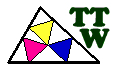1 a 20 20 a 40 40 a 60 60 a 80

 c1 Brocard points c2 Anticevian triangle c3 Tripole c4 Circle tangent to the circumcircle at a vertex and externally tangent to the incircle c5 Circle tangent to the circumcircle at a vertex and internally tangent to the incircle c6 Circle through two vertices and tangent to the incircle c7 Mannheim circle c8 Malfatti circles c9 Circle tangent to the circumcircle at a vertex and tangent to the opposite side c10 Triangle having its centroid at the incircle c11 Triangle knowing the vertex A, the circumcenter O and the symmedian point K c12 Equilateral triangle circumscribed to ABC c13 Triangle knowing the vertex A, the line r joining A and B and the ninecircle c c14 Triangle having its vertices on three concurrent lines r1, r2, r3 and as incenter I their concurrence point c15 Triangles ABC given A and B and such as the circle with diameter AB is tangent to the incircle c16 Triangles inscribed in ABC and similar to the pedal A'B'C' of a point P c17 Triangle ABC similar to A'B'C', C being given and A, B in two given lines r, s c18 Two points M, N isogonals with respect two triangles ABC and A'B'C' c19 Two tangent circles with the same radius, one of them with center A and the other through B and C c20 Triangle knowing ra, rb, rc perpendicular bisectors of the sides